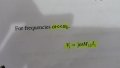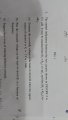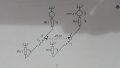# Inductive crosstalk voltage

#### ham3388

Joined Jul 3, 2012
97
Hello to all..
I'm facing difficulty in part a)

I'm not able to determine the current in circuit A to be able to put it in the below formula so to determine the crosstalk voltage in circiut BBelow are the diagram and the question.
Please guide me to find out the current since I don't have the value of the inductance in OHM#### ham3388

Joined Jul 3, 2012
97
I assumed since L1 compared to the sum of R1 and RL1 is very small so it can be negligted .then current =1/100=.01 A